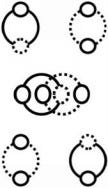# Music, Golf, and the Semiotics of ‘Meaning’

Nature’s ‘zero’ and ‘one.’ (Circle and Line)Photo by Cristina Anne Costello on Unsplash

Music gives a universal ‘clue’ as to the ‘meaning’ of golf (games in general).

## One and Two

Where you cannot have a winner without a loser (in golf). Meaning, in music, you cannot have a winner without a loser (you cannot prefer one type of music, or one piece of music, or one ‘artist’ in music, without at most, and at least, ‘one’ other).

That is, both music and golf require the number ‘two.’ And, also, and, therefore, the number ‘one.’ Proving mathematics is required for both.

This means we have to understand the numbers ‘one’ and ‘two’ if we want to understand the semiotics of ‘meaning.’ (The ‘meaning’ of ‘meaning.’) (The true ‘meaning’ of both music and golf).

## The Semiotics of Nature

So, right off the bat, we are involved in a semiotic exercise, because the diagram above stays ‘constant’ as the ‘numbers’ underneath change (are ‘variables’).

This is true, obviously, in music. And, in golf. That is, music and golf are semiotic exercises that help a human to understand ‘reality.’ (Semiotics, in general.) (The constant and the variable in general.)

Semiotics is the study of ‘signs’ and how ‘signs’ (zeroes and ones) are used, by humans (by Nature), to ‘interpret’ (experience) ‘reality’ (Nature in general).Semiotics (The Signs in Nature) (Zero and One)

## The Line and (the) Circle

Music uses a set of lines, and a set of circles (moving on the lines). And, golf, does the same. So we have to understand the ‘line’ and ‘circle’ if we want to understand music, golf, semiotics, and ‘meaning’ in general.

That is, the line and circle are diameter and circumference, literally, and figuratively, of an always-conserved (uber-basic) circle.

This explains (and exposes) why, and how, language (music) and games, are the same, even as, they are, obviously, different.

## The Circular (and Linear) Relationship

Where, again, there is a circular relationship between ‘same’ and ‘different.’

Music progresses from beginning to end, to leave an ‘impression’ on Nature (and, therefore, the human in general). Golf progresses from beginning to end, to leave an ‘impression’ on Nature (and, therefore, the human in general). Both are ‘expressions’ of Nature. (Both are expressions of ‘human’ Nature.) (Humans’ expressions of (and impressions on) Nature.)

Both depend on (what humans label) ‘time’ (in order to exist).

## Constant and Variable

So, our exercise, if we want to understand the ‘meaning’ of anything, is to understand ‘time.’ Because humans use ‘time’ as a ‘constant,’ and, also, a variable.

Time depends on complementary identity. Where complementary identity is the circular relationship between any X and Y (any zero and one). (Abstract and concrete.) (Abstractions, in general.)

This means the number ‘two’ is as basic as the number ‘one.’ Where the number ‘two’ is the correct ‘sign’ (articulation) for the number ‘one.’ Where humans can use ‘either’ in order to understand (interpret) (experience) ‘both.’

Because you cannot have one without two (or two without one). This is, obviously, because you cannot have a circumference (a circle) without a diameter (a line). And, therefore, ‘time’ is, from Nature’s point of view, ‘imaginary.’ (Non-existent.)

## X and (or) Y

Nature depends upon the(linear and) circular relationship of X and Y (life and death in any system) as does cognition, language, mathematics, music, and golf (time) (all systems and disciplines).X and Y (X or Y)

Where you cannot have ‘and’ without ‘or.’ And you cannot have ‘if’ without ‘then.’ Because you cannot have a circumference without a diameter. (Anything without the conservation of a circle.)

Proving ‘pi’ controls ‘reality.’ Where ‘pi’ is the correct name for ‘mind.’ (The search for ‘meaning’ in general.)

And the symbol, below, then, is the only correct representation (articulation) (observation) (‘sign’) for ‘reality.’ (Nature in general.) (Movement in general.) (Cognition in general.) (The human in general.)

Where ‘sound’ (movement in music) is an infinite circle. And ‘light’ (movement in golf ) is an infinite line. And they both, must, conserve (are completely dependent upon), an uber-simple circle (a.k.a. line).

Conservation of the circle is the core, and, therefore, the only dynamic in Nature. Exposing the true ‘meaning’ of ‘meaning.’ And the solution to the whole area of study called ‘semiotics.’